﻿ 高精度公共参数对轨道精度的影响分析文章快速检索 高级检索
 大地测量与地球动力学2020, Vol. 40Issue (6): 618-623  DOI: 10.14075/j.jgg.2020.06.014### 引用本文ZHENG Tao, XU Aigong, YANG Hu, et al. Analysis of Influence of High Precision Public Parameters on Orbit Accuracy[J]. Journal of Geodesy and Geodynamics, 2020, 40(6): 618-623.### Foundation support

National Natural Science Foundation of China, No.41504030, 41601501;National Key Research and Development Program of China, No.2016YFC0803102.

### 第一作者简介

ZHENG Tao, PhD, lecturer, majors in precision engineering survey, satellite navigation, data processing, E-mail:zhengtao210@126.com.

### 文章历史

1. 辽宁工程技术大学测绘与地理科学学院，辽宁省阜新市玉龙路88号，123000

1 联合定轨理论与方法 1.1 多GNSS数据处理观测方程

GNSS观测值中一般采用2种最基本的观测量, 即伪距和载波相位观测值。与单系统不同的是，多系统融合过程中由于各系统的观测值在卫星端与接收机端的延迟量不同，在融合过程中需要顾及系统间的信号延迟量，对于非GLONASS卫星此信号延迟量即系统间偏差，对于GLONASS卫星此信号延迟量为系统间偏差与接收机端频率偏差的统称。通常卫星端的系统间偏差可以被卫星钟差吸收，接收机端相位的系统间偏差可以被模糊度参数吸收，而接收机端伪距的系统间偏差则需要估计

GPS无论是在卫星星座、测站数量、测站覆盖范围还是系统稳定度、处理策略、产品类型多样化及产品精度方面，始终保持绝对的领先状态。因此多系统融合中，考虑各系统信号延迟问题时，仍然以GPS系统作为基准，将各系统间的不同信号延迟量均归算至相对于GPS的信号延迟量。需要说明的是，此时对多GNSS系统间的信号延迟量有一定的约束条件：1)所有接收机对于GPS的偏差值为0；2)所有接收机端对同一系统的偏差值和为0；3)所有接收机端对同一GLONASS频率的偏差值和为0。本文采用无电离层组合，则伪距和载波相位非差观测方程可描述为：

 $\left\{ \begin{array}{l} {\rm{PC}} = \rho + c({\rm{d}}{t_{\rm{r}}} - {\rm{d}}{t_{\rm{s}}}) + {d_{{\rm{ion}}}} + {d_{{\rm{trop}}}} + \\ {\kern 1pt} {\kern 1pt} {\kern 1pt} {\kern 1pt} {\kern 1pt} {\kern 1pt} {\kern 1pt} {\kern 1pt} {\kern 1pt} {\kern 1pt} {\kern 1pt} {\kern 1pt} {\kern 1pt} c{\delta _{{\rm{tg}}}} + {\varepsilon _p}\\ {\rm{LC}} = \rho + c({\rm{d}}{t_{\rm{r}}} - {\rm{d}}{t_{\rm{s}}}) - {d_{{\rm{ion}}}} + {d_{{\rm{trop}}}} + \\ {\kern 1pt} {\kern 1pt} {\kern 1pt} {\kern 1pt} {\kern 1pt} {\kern 1pt} {\kern 1pt} {\kern 1pt} {\kern 1pt} {\kern 1pt} {\kern 1pt} {\kern 1pt} {\kern 1pt} c{\delta _{{\rm{tg}}}} + N\lambda + {\varepsilon _\varPhi } \end{array} \right.$ (1)

1.2 动力学模型与处理策略

 $\bar a = {\bar a_{\rm{g}}} + {\bar a_{{\rm{ng}}}} + {\bar a_{{\rm{emp}}}}$ (2)表 1 多GNSS联合定轨处理策略 Tab. 1 Multi-GNSS joint orbit determination model表 2 多GNSS联合定轨动力学模型 Tab. 2 Multi-GNSS joint orbit determination dynamics model
1.3 公共参数贡献量理论分析

 ${\mathit{\boldsymbol{V}}_1} = {\mathit{\boldsymbol{L}}_1} - \left[ {\begin{array}{*{20}{l}} {{\mathit{\boldsymbol{A}}_1}}&{{\mathit{\boldsymbol{B}}_1}} \end{array}} \right]{\left[ {\begin{array}{*{20}{l}} {{\mathit{\boldsymbol{X}}_1}}\\ \mathit{\boldsymbol{X}} \end{array}} \right]^{\rm{T}}}$ (3)

 ${\mathit{\boldsymbol{U}}_1} = {\mathit{\boldsymbol{L}}_1} - (\mathit{\boldsymbol{I}} - {\mathit{\boldsymbol{J}}_1}){\mathit{\boldsymbol{B}}_1}\mathit{\boldsymbol{X}}$ (4)

 ${\mathit{\boldsymbol{J}}_1} = {\mathit{\boldsymbol{A}}_1}{(\mathit{\boldsymbol{A}}_1^{\rm{T}}{\mathit{\boldsymbol{P}}_1}{\mathit{\boldsymbol{A}}_1})^{ - 1}}\mathit{\boldsymbol{A}}_1^{\rm{T}}{\mathit{\boldsymbol{P}}_1}$ (5)

 $\mathit{\boldsymbol{\hat X}} = {(\mathit{\boldsymbol{C}}_1^{\rm{T}}{\mathit{\boldsymbol{P}}_1}{\mathit{\boldsymbol{C}}_1})^{ - 1}}(\mathit{\boldsymbol{C}}_1^{\rm{T}}{\mathit{\boldsymbol{P}}_1}{\mathit{\boldsymbol{L}}_1})$ (6)

 $\left[ {\begin{array}{*{20}{l}} {{\mathit{\boldsymbol{U}}_1}}\\ {{\mathit{\boldsymbol{U}}_2}} \end{array}} \right] = \left[ {\begin{array}{*{20}{l}} {{\mathit{\boldsymbol{L}}_1}}\\ {{\mathit{\boldsymbol{L}}_2}} \end{array}} \right] - \left[ {\begin{array}{*{20}{l}} {{\mathit{\boldsymbol{C}}_1}}\\ {{\mathit{\boldsymbol{C}}_2}} \end{array}} \right]{\mathit{\boldsymbol{X}}^\prime }$ (7)

 ${\mathit{\boldsymbol{\hat X}}^\prime } = {(\mathit{\boldsymbol{C}}_1^{\rm{T}}{\mathit{\boldsymbol{P}}_1}{\mathit{\boldsymbol{C}}_1} + \mathit{\boldsymbol{C}}_2^{\rm{T}}{\mathit{\boldsymbol{P}}_2}{\mathit{\boldsymbol{C}}_2})^{ - 1}}(\mathit{\boldsymbol{C}}_1^{\rm{T}}{\mathit{\boldsymbol{P}}_1}{\mathit{\boldsymbol{L}}_1} + \mathit{\boldsymbol{C}}_2^{\rm{T}}{\mathit{\boldsymbol{P}}_2}{\mathit{\boldsymbol{L}}_2})$ (8)

 ${\rm{d}}\mathit{\boldsymbol{\hat X}} = {\mathit{\boldsymbol{\hat X}}^\prime } - \mathit{\boldsymbol{\hat X}}$ (9)

2 实验及结果分析 2.1 测站选择图 1 测站分布示意图 Fig. 1 Sketch of stations' distribution

2.2 实验结果分析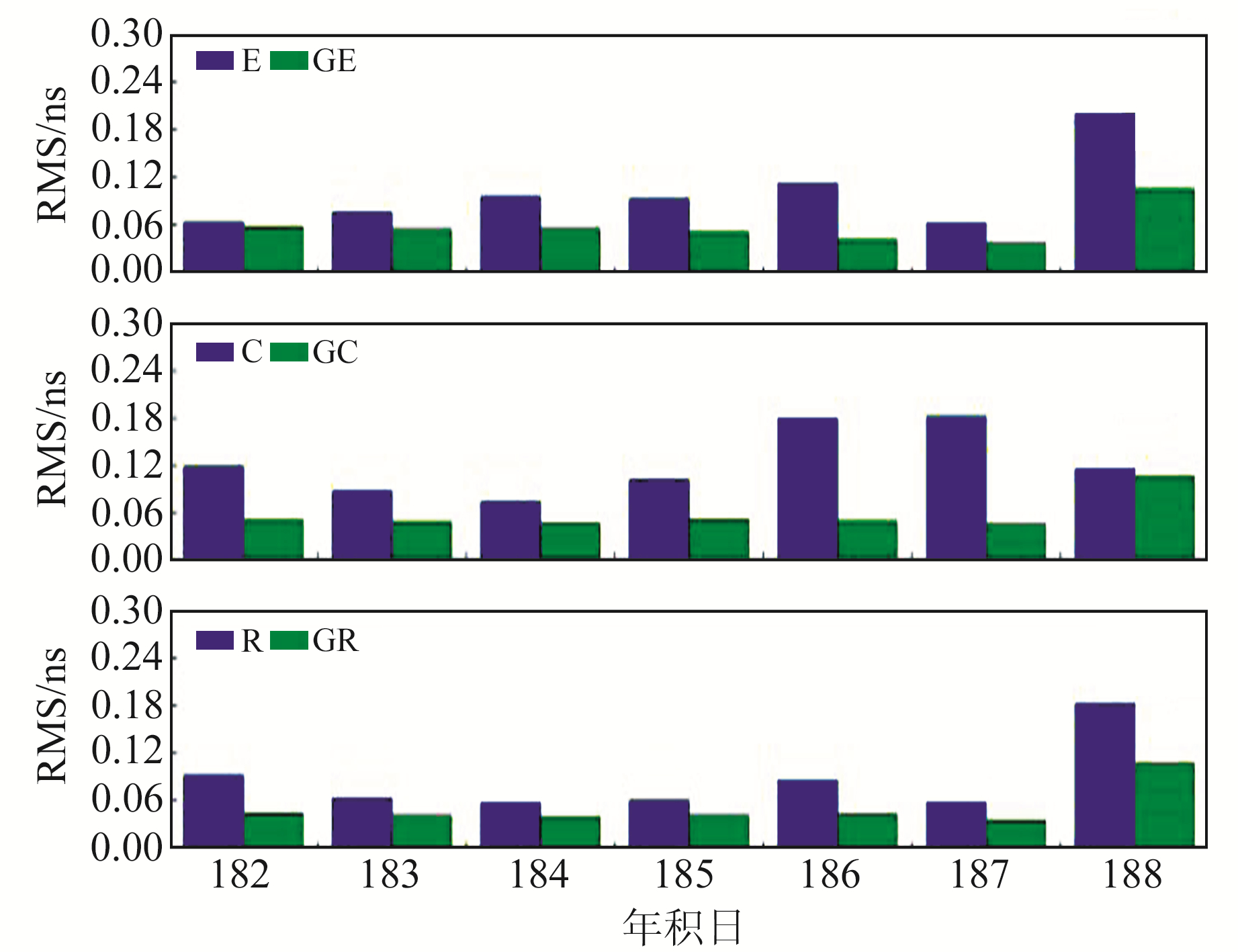图 2 接收机钟差RMS Fig. 2 RMS of receiver clock error表 3 公共参数精度提升量 Tab. 3 Improvement of public parameters by GPS common parameters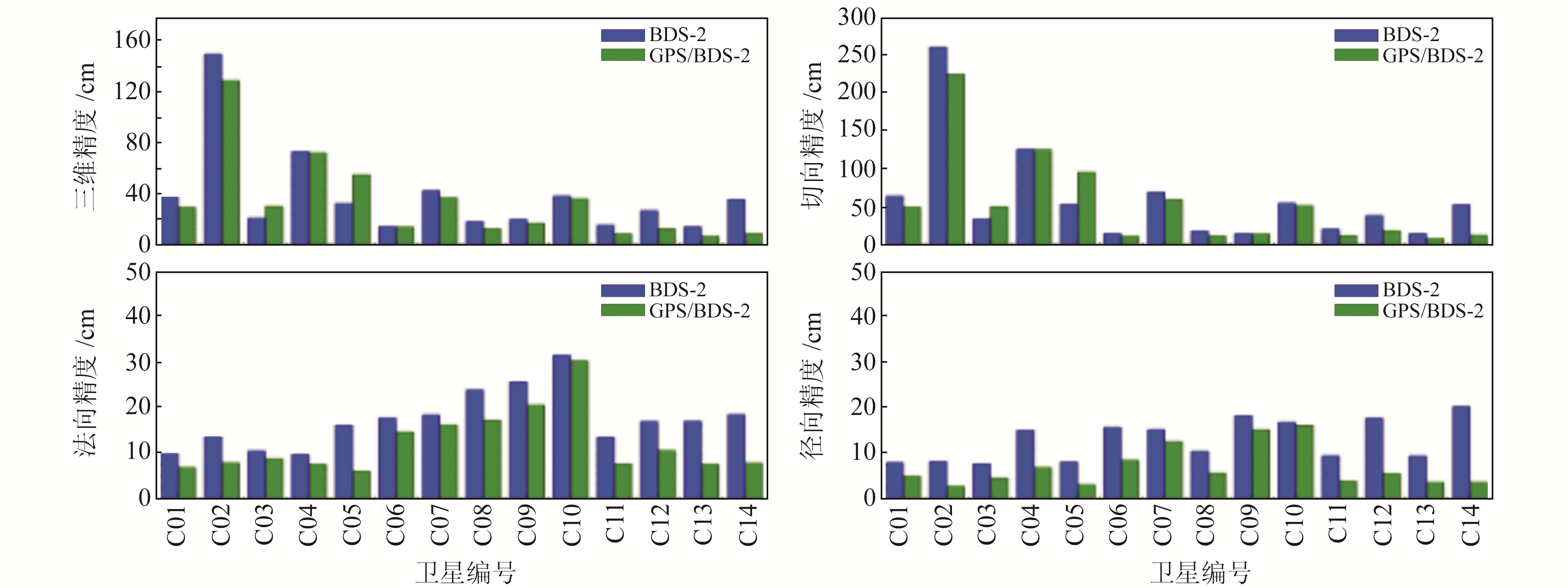图 3 BDS-2与GPS/BDS-2定轨的三维平均、切向、法向、径向的精度对比 Fig. 3 Comparison of 3-D average, tangential, normal and radial accuracy of BDS-2 and GPS/BDS-2 orbit determination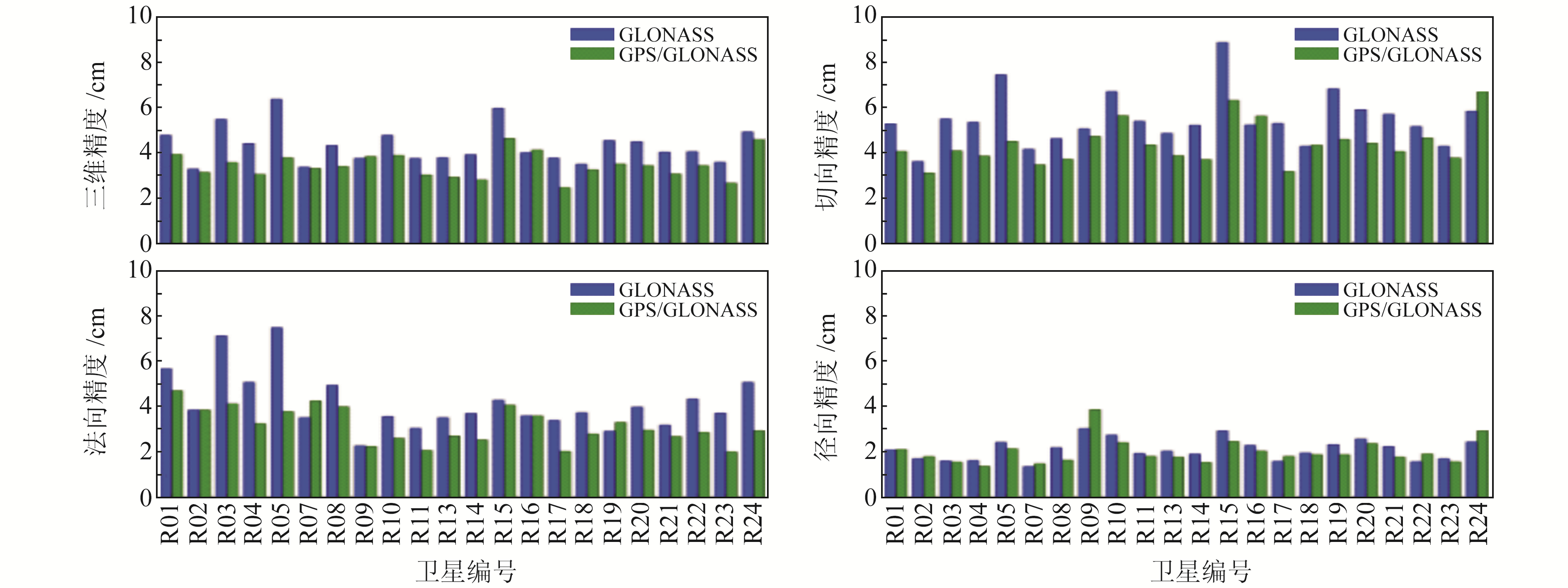图 4 GLONASS与GPS/GLONASS定轨的三维平均、切向、法向、径向的精度对比 Fig. 4 Comparison of 3-D average, tangential, normal and radial accuracy of GLONASS and GPS/GLONASS orbit determination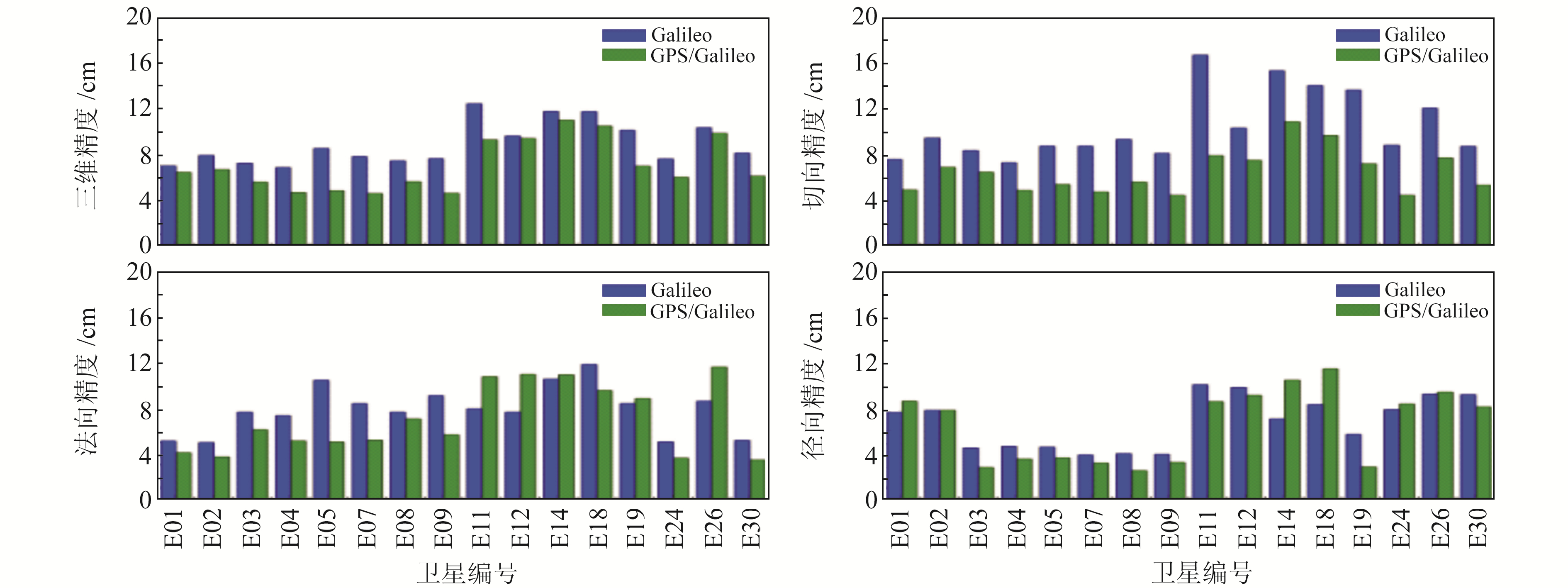图 5 Galileo与GPS/Galileo定轨的三维平均、切向、法向、径向的精度对比 Fig. 5 Comparison of 3D average, tangential, normal and radial accuracy of Galileo and GPS/Galileo orbit determination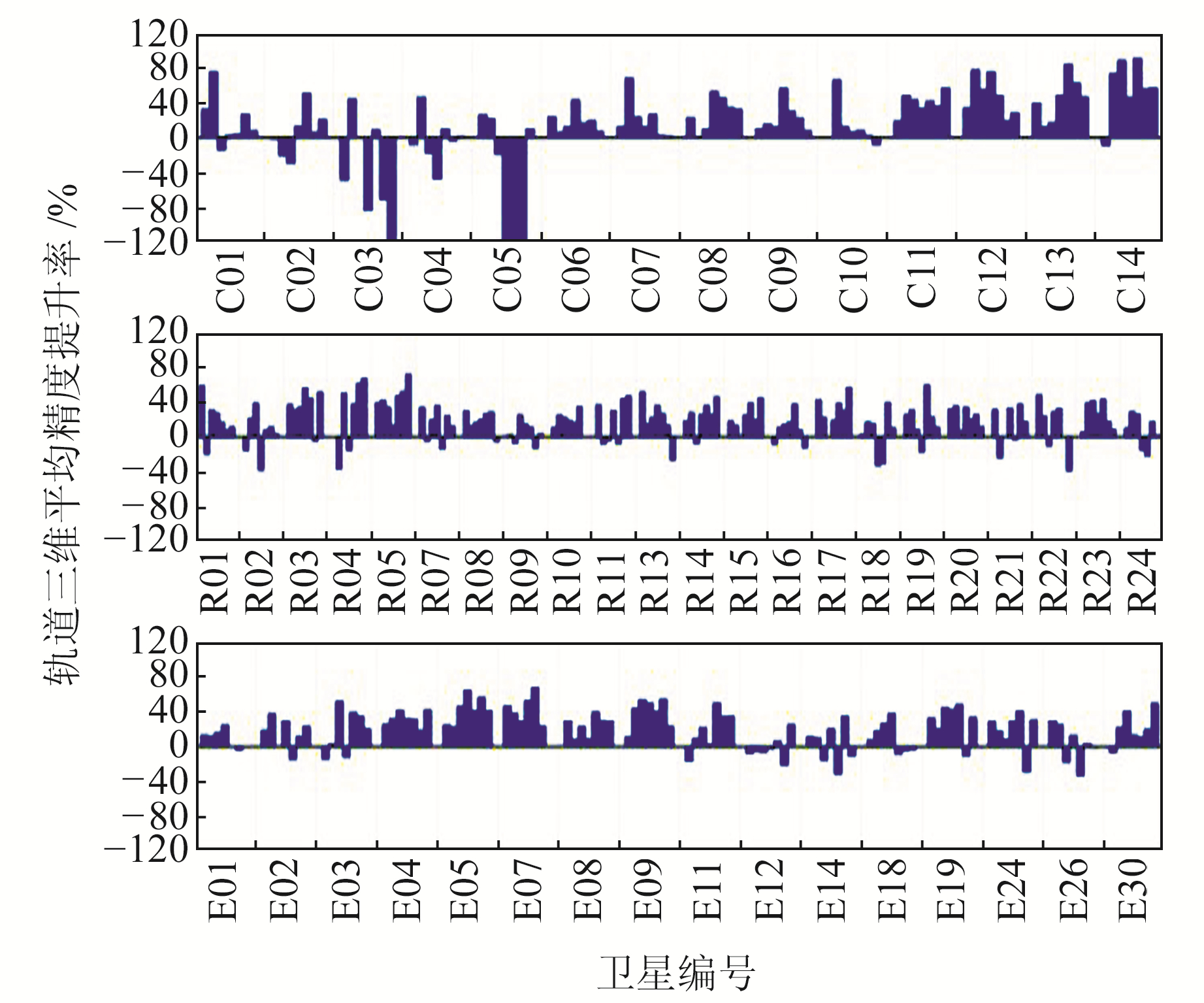图 6 GPS对各系统各天轨道三维平均精度的提升量 Fig. 6 GPS 3D average accuracy improvement of each system's orbits表 4 GPS公共参数对轨道精度的提升量/% Tab. 4 Improvement of orbit accuracy by GPS common parameters/%

1) 由于BDS-2、GLONASS、Galileo单系统解算的公共参数精度不高，在进行与GPS联合定轨时，这3个系统对于公共参数的观测值数量越少，在进行法方程求解时对于解算公共参数的影响越小，联合定轨后相关的参数精度提升量越大。

2) GPS高精度公共参数对于BDS-2的GEO卫星在切向上的轨道精度无明显提升，法向与径向有所提高。在切向上，GEO卫星精度呈现一种随机性，即与联合定轨精度BDS-2单系统定轨精度相比较，部分卫星轨道精度有所提高(如C01、C02)，部分卫星轨道精度有所下降(如C03、C05)，部分卫星轨道精度基本不变(如C04)。其根本原因可能是由GEO卫星的轨道特性决定的，GEO卫星相对于各个地面跟踪站基本不变，轨道动力学模型精度差、几何观测信息差，导致其本身定轨精度差。

3 结语

1) 对于BDS-2、GLONASS、Galileo等地面跟踪站数量少、分布不均匀或者卫星星座不完整的导航定位系统，加入GPS系统进行联合定轨可以改善公共参数的精度，最终体现为各系统卫星轨道精度的提高。

2) BDS-2由于其卫星轨道的特殊性，联合求解的公共参数对BDS-2卫星轨道精度的影响不可一概而论。联合求解后，仅GEO卫星的切向精度呈现随机性，但GEO卫星的法向与径向精度均有提升；IGSO、MEO卫星轨道精度也有提升，其中MEO卫星轨道精度提升量达到60%以上。

3) GLONASS与Galileo使用GPS解算的公共参数进行联合定轨，较其单系统定轨精度均有一定程度的提升，分别提升了19.80%、21.23%。由于GLONASS与Galileo单系统定轨精度较高，因此GPS公共参数对其提升无BDS-2明显。

  杨元喜. 综合PNT体系及其关键技术[J]. 测绘学报, 2016, 45(5): 505-510 (Yang Yuanxi. Concepts of Comprehensive PNT and Related Key Technologies[J]. Acta Geodaetica et Cartographica Sinica, 2016, 45(5): 505-510) (0)  杨元喜, 李金龙, 王爱兵, 等. 北斗区域卫星导航系统基本导航定位性能初步评估[J]. 中国科学:地球科学, 2014, 44(1): 72-81 (Yang Yuanxi, Li Jinlong, Wang Aibing., et al. Preliminary Assessment of the Navigation and Positioning Performance of Beidou Regional Navigation Satellite System[J]. Science China: Earth Sciences, 2014, 44(1): 72-81) (0)  杨元喜, 许扬胤, 李金龙, 等. 北斗三号系统进展及性能预测——试验验证数据分析[J]. 中国科学:地球科学, 2018, 48(5): 584-594 (Yang Yuanxi, Xu Yangyin, Li Jinlong, et al. Progress and Performance Evaluation of Beidou Global Navigation Satellite System: Data Analysis Based on BDS-3 Demonstration System[J]. Science China: Earth Sciences, 2018, 48(5): 584-594) (0)  李敏, 施闯, 赵齐乐, 等. 多模全球导航卫星系统联合精密定轨[J]. 测绘学报, 2011, 40: 26-30 (Li Min, Shi Chuang, Zhao Qile., et al. Multi-GNSS Precision Orbit Determination[J]. Acta Geodaetica et Cartographica Sinica, 2011, 40(S1): 26-30) (0)  刘伟平, 郝金明, 李建文, 等. 多GNSS融合的北斗卫星精密定轨[J]. 测绘学报, 2014, 43(11): 1 132-1 138 (Liu Weiping, Hao Jinming, Li Jianwen, et al. Multi-GNSS Joint Precise Orbit Determination of Beidou Navigation Satellite System[J]. Acta Geodaetica et Cartographica Sinica, 2014, 43(11): 1 132-1 138) (0)  王乐, 黄观文, 张勤, 等. 基于区域监测站的BDS定轨策略分析[J]. 大地测量与地球动力学, 2018, 38(5): 497-509 (Wang Le, Huang Guanwen, Zhang Qin, et al. Analysis of Strategy of BDS Satellite Orbit Determination Using Regional Monitoring Statioin[J]. Journal of Geodesy and Geodynamics, 2018, 38(5): 497-509) (0)  党亚民, 张龙平, 陈俊勇. 多GNSS系统精密定轨ISB/IFB估计及特性分析[J]. 武汉大学学报:信息科学版, 2018, 43(12): 2 079-2 138 (Dang Yamin, Zhang Longping, Chen Junyong, et al. ISB/IFB Estimation and Characteristic Analysis with Multi-GNSS Precise Orbit Determination[J]. Geomatics and Information Science of Wuhan University, 2018, 43(12): 2 079-2 138) (0)  杨徐, 祝会忠, 徐爱功, 等. BDS/GPS联合精密定轨理论与精度分析[J]. 导航定位学报, 2018, 6(2): 87-92 (Yang Xu, Zhu Huizhong, Xu Aigong, et al. Analysis on Theory and Accuracy of BDS/GPS Precise Orbit Determination[J]. Journal of Navigation and Positioning, 2018, 6(2): 87-92) (0)  Guo J, Xu X L, Zhao Q L, et al. Precise Orbit Determination for Quad-Constellation Satellites at Wuhan University: Strategy, Result Validation, and Comparison[J]. Cross Mark, 2016, 90: 143-159 (0)  张睿, 杨元喜, 张勤, 等. BDS/GPS联合定轨的贡献分析[J]. 武汉大学学报:信息科学版, 2017, 42(5): 600-608 (Zhang Rui, Yang Yuanxi, Zhang Qin., et al. Contribution Analysis of BDS/GPS Combined Orbit Determination[J]. Geomatics and Information Science of Wuhan University, 2017, 42(5): 600-608) (0)  张龙平, 党亚民, 成英燕, 等. 北斗GEO/IGSO/MEO卫星定轨地面站构型影响分析及其优化[J]. 测绘学报, 2016, 45: 82-92 (Zhang Longping, Dang Yamin, Cheng Yingyan, et al. Analysis and Optimization on BDS GEO/IGSO/MEO Ground Monitoring Stations Configuration for Determining GNSS Orbit[J]. Acta Geodaetica et Cartographica Sinica, 2016, 45(S2): 82-92) (0)  计国锋, 杨志强, 贾小林. MGEX和IGMAS的多系统轨道和钟差产品精度分析[J]. 大地测量与地球动力学, 2019, 39(1): 13-19 (Ji Guofeng, Yang Zhiqiang, Jia Xiaolin, et al. Precise Orbit and Clock Products for Multi-GNSS System from MGEX and iGMAS[J]. Journal of Geodesy and Geodynamics, 2019, 39(1): 13-19) (0)  Zhang R, Tu R, Liu J H, et al. Performance of Galileo: Global Coverage, Precise Orbit Determination, and Precise Positioning[J]. Advance in Space Research, 2019, 42(3): 1-35 (0)
Analysis of Influence of High Precision Public Parameters on Orbit Accuracy
ZHENG Tao1XU Aigong1     YANG Hu1     TANG Longjiang1
1. School of Mapping and Geographical Science, Liaoning Technical University, 88 Yulong Road, Fuxin 123000, China
Abstract: Due to the small number and uneven distribution of tracking stations of BDS-2, GLONASS, Galileo satellites, it is necessary to obtain high-precision common parameters with GPS in the actual BDS-2/GLONASS/Galileo orbit determination process to improve orbit accuracy. In order to optimize the data processing strategy of joint orbit determination, it is necessary to analyze the influence of the common parameters in the joint orbit determination on the orbit. This paper analyzes the contribution of common station coordinates, tropospheric and receiver clock parameters, to multi-system joint orbit determination in the non-ionosphere combination from the theoretical point of view. Finally, we carry out single-system and multi-system joint orbit determination experiments. The experimental results show that the high-precision common parameters have a significant effect on the accuracy of the orbit determination of each single system. The accuracy of the orbit accuracy of the IGSO of BDS-2, GLONASS, and Galileo can be improved by almost 20%, and the orbit accuracy of the MEO of BDS-2 can be improved by almost 60%.
Key words: multi-system; joint; public parameters; orbit